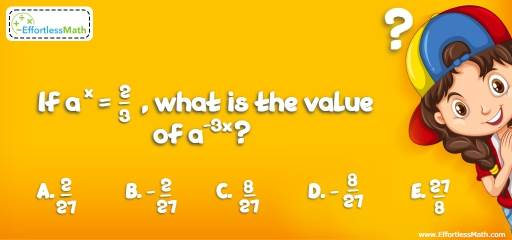# Number Properties Puzzle – Challenge 14

This is a great math challenge to tease your brain and sharpen your basic math skills. This puzzle is for you if you love critical thinking challenges. Let's challenge your brain!## Challenge:

If $$a^{x }= \frac{2}{3}$$, what is the value of $$a^{-3x}$$?

A- $$\frac{2}{27}$$

B- $$-\frac{2}{27}$$

C- $$\frac{8}{27}$$

D- $$-\frac{8}{27}$$

E- $$-\frac{27}{8}$$

### The Absolute Best Book to challenge your Smart Student!

$$a^{x} = \frac{2}{3 }→ a^{-3x} = (\frac{2}{3})^{-3} = \frac{1}{(\frac{2}{3})^{3}} = \frac{1}{\frac{8}{27}} =\frac{ 27}{8}$$

The Absolute Best Books to Ace Algebra

### What people say about "Number Properties Puzzle – Challenge 14 - Effortless Math: We Help Students Learn to LOVE Mathematics"?

No one replied yet.

X
45% OFF

Limited time only!

Save Over 45%

SAVE $40 It was$89.99 now it is \$49.99